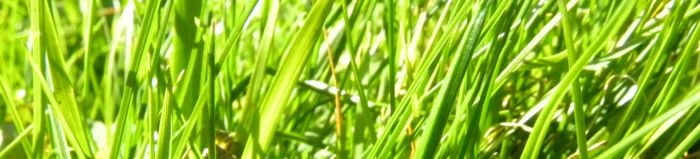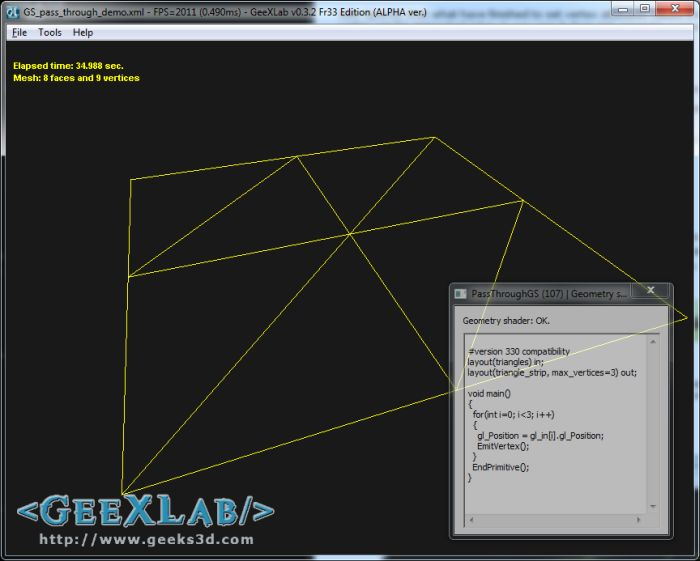# Simple Introduction to Geometry Shaders in GLSL (Part 1)UPDATE: Part 2 is available HERE.

## 1 – Geometry shaders overview

The geometry shader (or GS) stage is located after the vertex shader (or tessellation if used) one and before the rasterizer and pixel shader ones (check out this article for more details). The geometry shader is the latest stage of the 3D pipeline that can manipulate geometry. The geometry shader acts on a complete primitive (triangle or line): it can modify existing primitives, it can insert (create) new primitives, it can remove (destroy) existing primitives.

```glCreateShader(GL_GEOMETRY_SHADER).
```

We’re going to see two very simple uses of the geometry shader stage in GLSL: a pass-through GS (part 1) and a geometry doubler (part 2).

## 2 – Pass-through geometry shader

I think it’s the simplest geometry shader we can find: the only thing it does is to send the input primitives (a triangle) to the rasterizer (the stage right after the GS and just before the pixel shader stage) without transformation. In a word, it’s a useless GS. But it’s perfect to see the principle of its working.

The demo is available at the end of the article.

```uniform mat4 viewMatrix;
uniform mat4 projectionMatrix;
uniform mat4 modelMatrix;

void main()
{
vec4 world_pos = modelMatrix * gl_Vertex;
vec4 view_pos = viewMatrix * world_pos;
gl_Position = projectionMatrix * view_pos;
}
```

```#version 330 compatibility
layout(triangles) in;
layout(triangle_strip, max_vertices=3) out;

void main()
{
for(int i=0; i<3; i++)
{
gl_Position = gl_in[i].gl_Position;
EmitVertex();
}
EndPrimitive();
}
```

```void main(void)
{
gl_FragColor = vec4(1.0, 1.0, 0.0, 1.0);
}
```

Let's quickly explain the GS.

```#version 330 compatibility
```

This GLSL program comes from a GeeXLab demo and GeeXLab uses the ARB compatibility mode in order to mix OpenGL 2 and OpenGL 3 and 4 functionalities.

```layout(triangles) in;
```

tells OpenGL that the input primitive is a triangle (made up of 3 vertices).

```layout(triangle_strip, max_vertices=3) out;
```

tells OpenGL that the output primitive is also a triangle (3 vertices). Only triangle_strip is supported (or line_strip if you need lines instead of triangles).

```    gl_Position = gl_in[i].gl_Position;
```

gl_in is a built-in array (the size of the array is related to the number of vertices of the input primitive, here 3) that contains several variables, including the vertex position gl_Position.

```    EmitVertex();
```

tells OpenGL that what have finished to set vertex attibutes values (here only the position) and that a new vertex can be generated (okay in our case it does not generate a new vertex, since vertices already exist).

```  EndPrimitive();
```

tells OpenGL we have finished to create a primitive (in our case a triangle).

I hope this first example has somewhat demystified the geometry shader stage. In the second part, we'll see a more interesting use of the GS.

As usual, if you notice mistakes or have some interesting information to share, the comments section if for you!

## 3 - The Demo

 The GeeXLab demo is available in the GLSL_Geometry_Shader/ folder of GeeXLab code sample pack: [download#40#image] This demo requires GeeXLab 0.3.2+ (GS support has been added in the 0.3.x branch of GeeXLab).## 4 thoughts on “Simple Introduction to Geometry Shaders in GLSL (Part 1)”

1.Leith Bade

You can do cool stuff with GS. I was able to render thick lines by using geometry shader to convert a line primitive into a triangle strip.

2.GPUToaster

Nice Explanation.!!

3.Sylph

Is it possible to have input like :

vertex
(
position(x,y,z) = 3 floats * 4 bytes,
color(r,g,b) = 3 bytes;
color(alpha) = 1 byte – which side of cube is visible using bitfield (one bit per side, 0 = not visible, 1 = visible)
)

Then in geometry shader – take one primitive and generate a colored voxel cube of max 6 sides and min 1 side depending on precomputed bitfield value(color-alpha byte), with normals for each side for light?

And it is possible to store generated triangles into a VBO?

4.Jack

I hate to nit-pick, but this part isn’t clear at all:

EmitVertex();
tells OpenGL that what have finished to set vertex attibutes values (here only the position) and that a new vertex can be generated (okay in our case it does not generate a new vertex, since vertices already exist).

I’m not quite sure what you mean to say, but maybe it should be:
“This tells OpenGL that (after geometry shader processing,) vertex positions will be set. Also that new vertices can be generated, (even though the example shown here generates no new vertices.)

I’m still learning about geometry shaders, so I take no responsibility for the technical accuracy of my attempted edit, only for the grammar.

Thanks for sharing your experience with us!